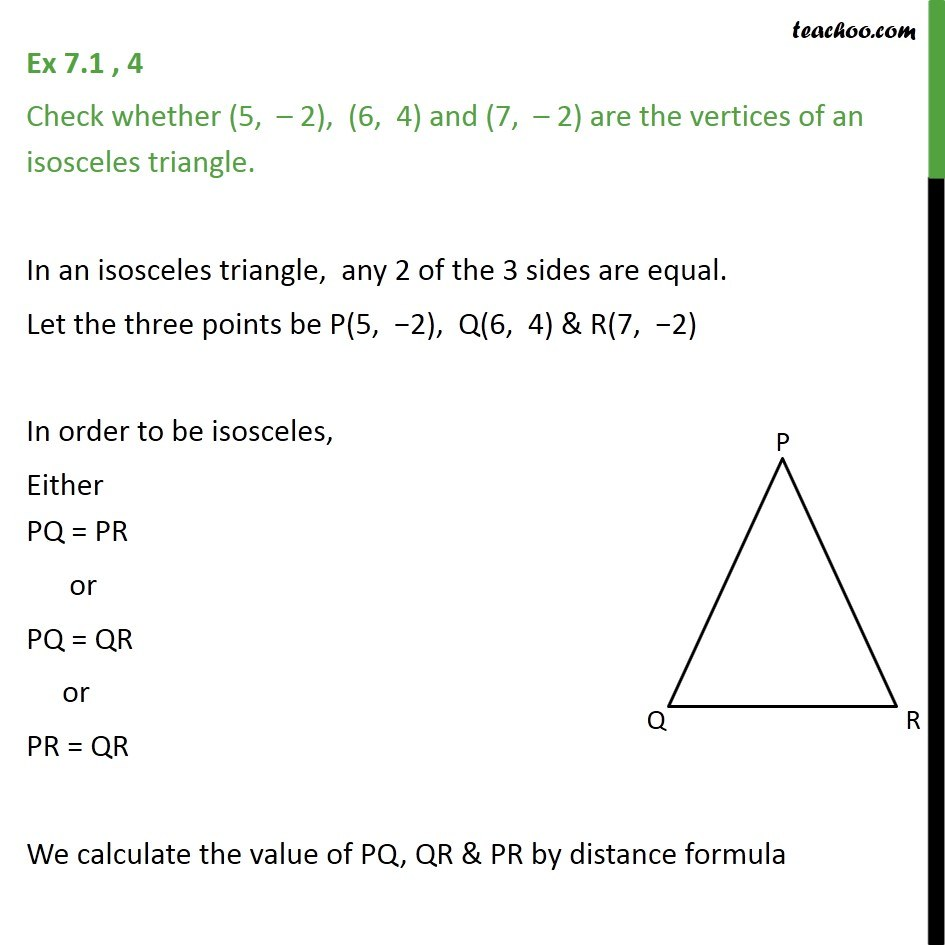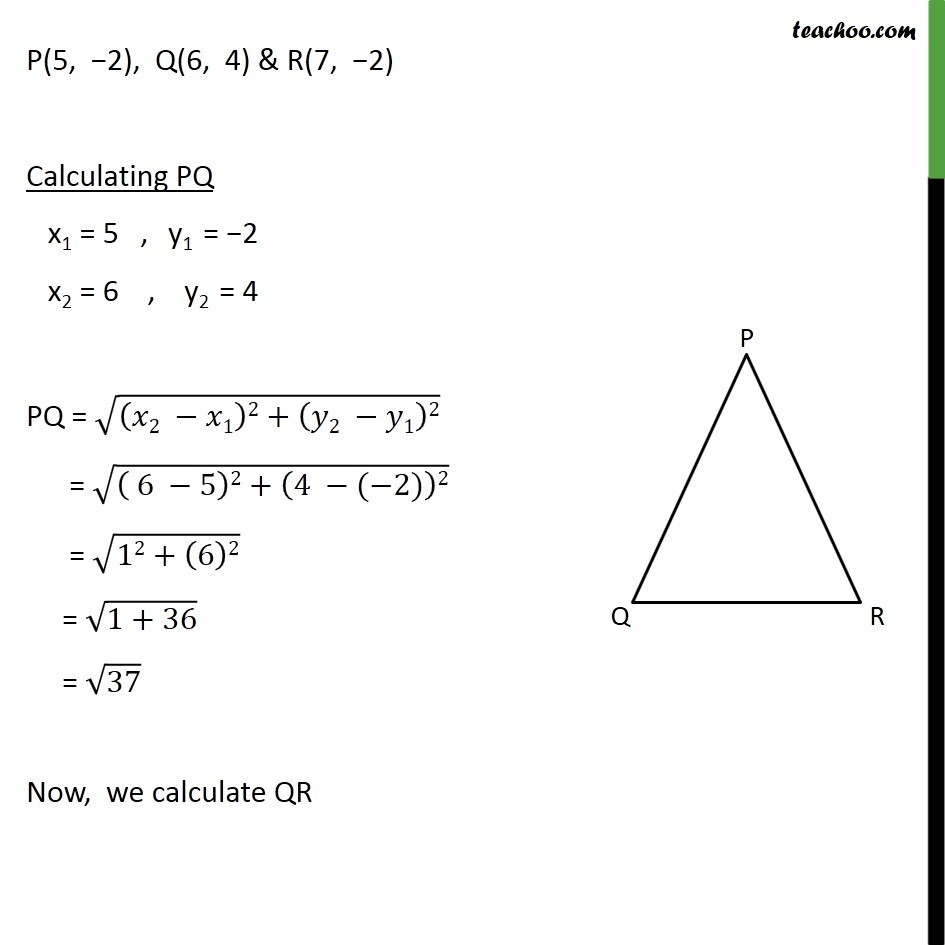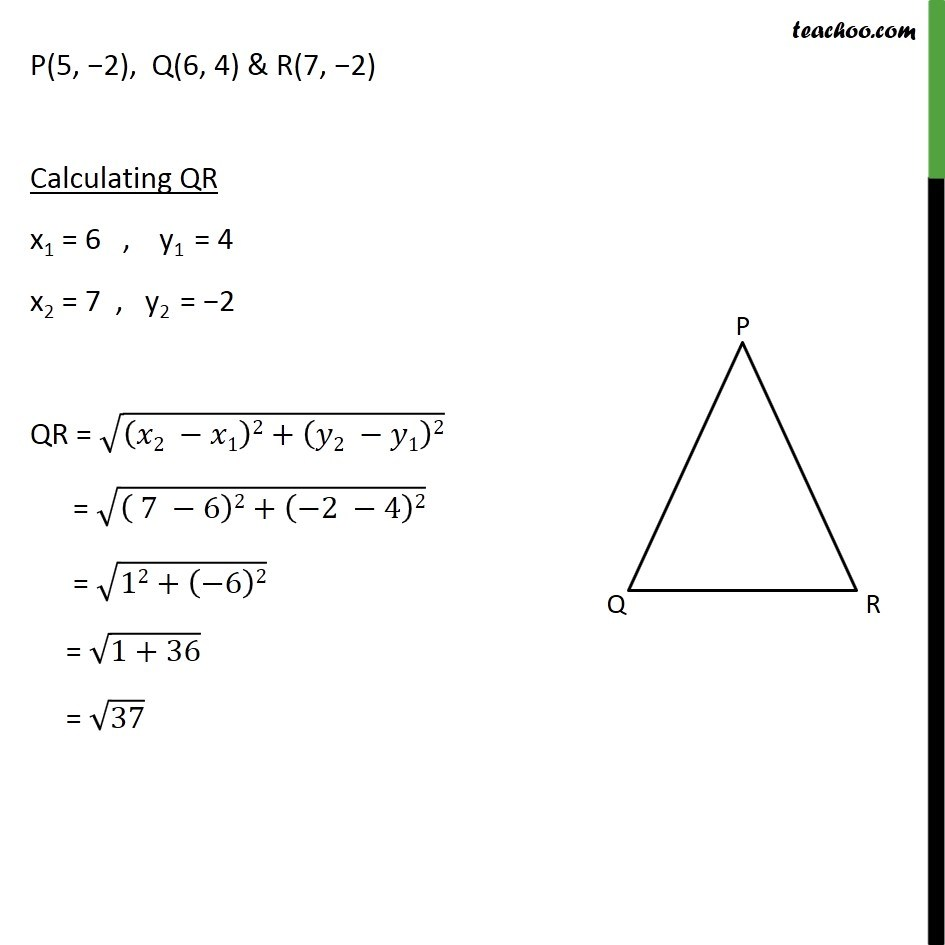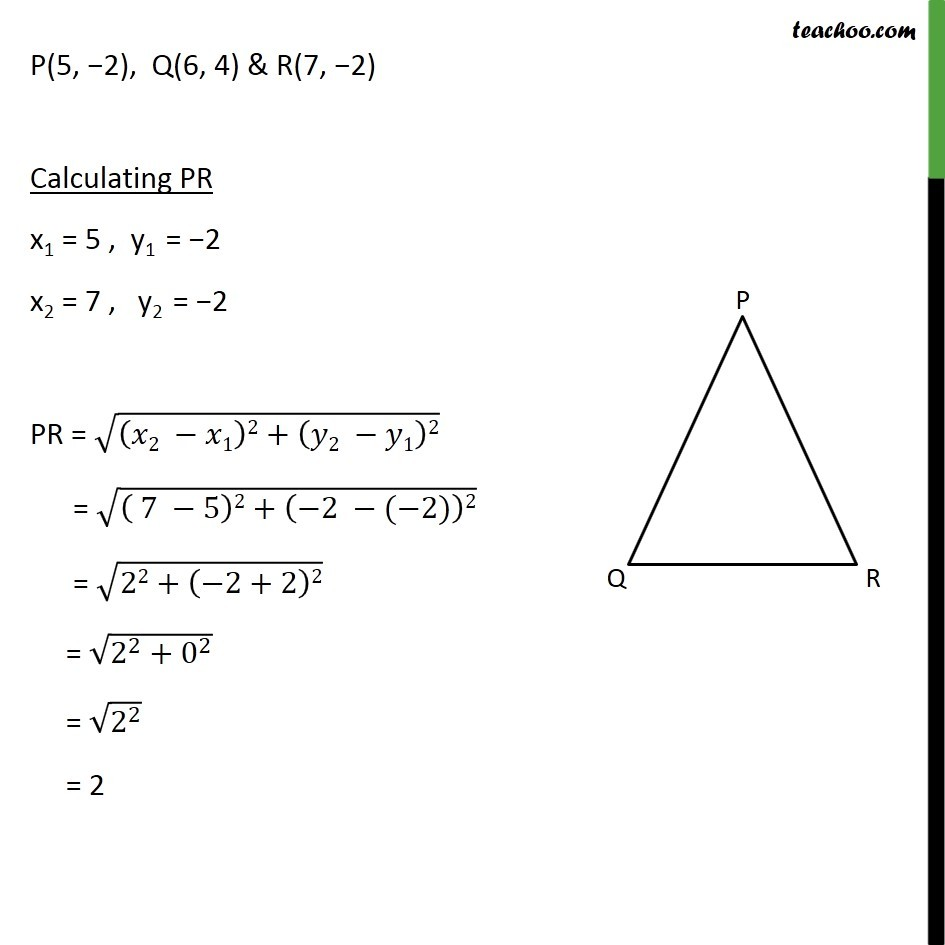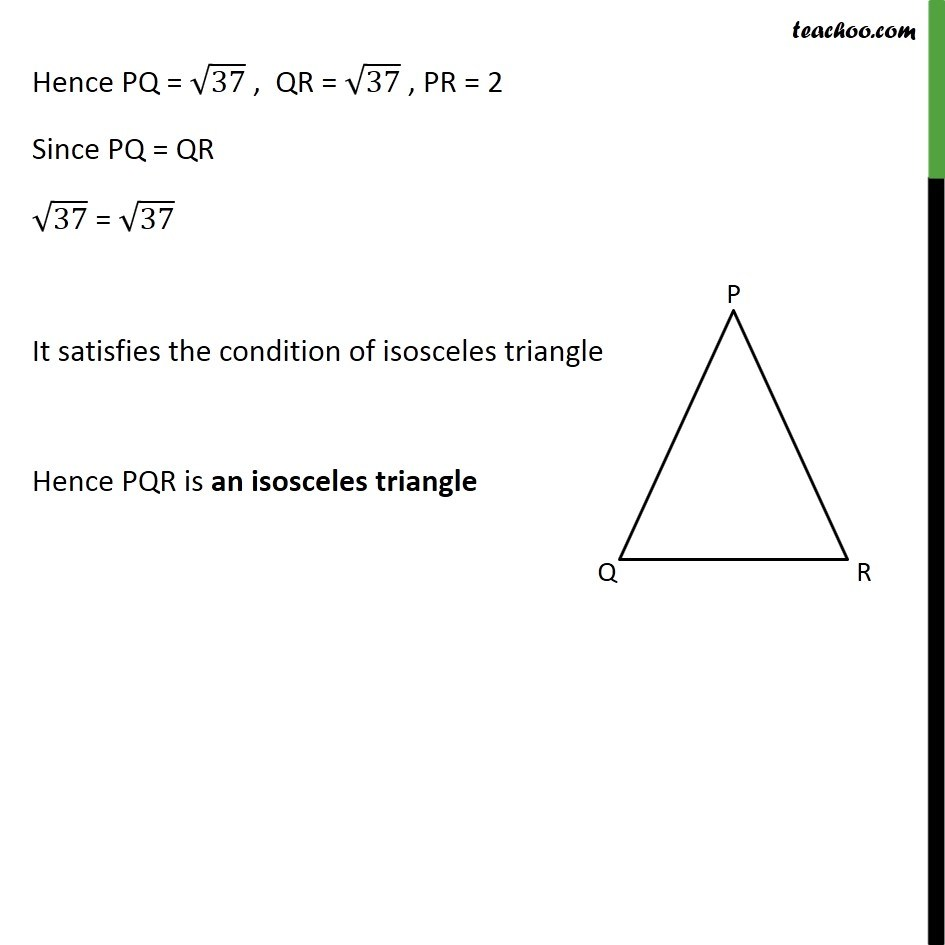1. Chapter 7 Class 10 Coordinate Geometry
2. Serial order wise
3. Ex 7.1

Transcript

Ex 7.1 , 4 Check whether (5, – 2), (6, 4) and (7, – 2) are the vertices of an isosceles triangle. In an isosceles triangle, any 2 of the 3 sides are equal. Let the three points be P(5, −2), Q(6, 4) & R(7, −2) In order to be isosceles, Either PQ = PR or PQ = QR or PR = QR We calculate the value of PQ, QR & PR by distance formula P(5, −2), Q(6, 4) & R(7, −2) Calculating PQ x1 = 5 , y1 = −2 x2 = 6 , y2 = 4 PQ = √((𝑥2 −𝑥1)2+(𝑦2 −𝑦1)2) = √(( 6 −5)2+(4 −(−2))2) = √(12+(6)2) = √(1+36) = √37 Now, we calculate QR P(5, −2), Q(6, 4) & R(7, −2) Calculating QR x1 = 6 , y1 = 4 x2 = 7 , y2 = −2 QR = √((𝑥2 −𝑥1)2+(𝑦2 −𝑦1)2) = √(( 7 −6)2+(−2 −4)2) = √(12+(−6)2) = √(1+36) = √37 P(5, −2), Q(6, 4) & R(7, −2) Calculating PR x1 = 5 , y1 = −2 x2 = 7 , y2 = −2 PR = √((𝑥2 −𝑥1)2+(𝑦2 −𝑦1)2) = √(( 7 −5)2+(−2 −(−2))2) = √(22+(−2+2)2) = √(2^2+0^2 ) = √(2^2 ) = 2 Hence PQ = √37 , QR = √37 , PR = 2 Since PQ = QR √37 = √37 It satisfies the condition of isosceles triangle Hence PQR is an isosceles triangle

Ex 7.1